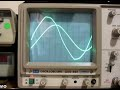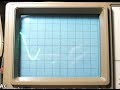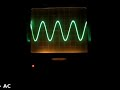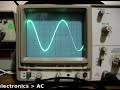# Planet Arduino

Lug
15

## Education – Introduction to Alternating Current – part two

#### AC, alternating current, CRO, education, electronics tutorial, learning electronics, oscilloscope, phase, phase shift, reactance, resistor, resistor-capacitor, sine wave, tronixstuff, tutorial, voltage dividerCommenti disabilitati su Education – Introduction to Alternating Current – part two

Hello everyone

Today we are going to continue exploring alternating current, with regards to how resistors and capacitors deal with AC. This chapter is part two, chapter one is here.

To help with the explanations, remember this diagram:That is, note that there are three possible voltage values, Vpp, Vp and Vrms. Moving on. Alternating current flows through various components just like direct current. Let’s examine some components and see.

First, the resistor. It operates in the same way with AC as it does DC, and the usual calculations apply with regards to Ohm’s law, dividing voltage and so on. However you must keep in mind the type of voltage value. For example, 10Vrms + 20Vpp does NOT equal 30 of anything. But we can work it out. 20Vpp is 10Vp,  which is 7.07Vrms… plus 10Vrms = 17.07Vrms. Therefore, 10Vrms + 20Vpp = 17.07Vrms.

Furthermore, when using Ohm’s law, or calculating power, the result of your equation must always reflect the type of voltage used in the calculations. For example:Next, the capacitor. Capacitors oppose the flow of alternating current in an interesting way – in simple terms, the greater the frequency of the current, the less opposition to the current. However, we call this opposition reactance, which is measured in ohms. Here is the formula to calculate reactance:the result Xc is measured in Ohms, f is frequency is Hertz, and C is capacitance in Farads. Here are two examples – note to convert the value of the capacitor back to FaradsAlso consider if you have identical frequencies, a smaller capacitor will offer a higher resistance than a larger capacitor. Why is this so? A smaller capacitor will reach the peak voltages quicker as it charges in less time (as it has less capacitance); wheras a larger capacitor will take longer to charge and reach the peak voltage, therefore slowing down the current flow which in turn offers a higher reactance.

Resistors and capacitors can also work together as an AC voltage divider. Consider the following schematic:As opposed to a DC voltage divider, R2 has been replaced with C1, the 0.1 uF capacitor. In order to calculate Vout, we will need the reactance of C1 – and subsitute that value for R2:However, once the voltage has been divided, Vout has been transformed slightly – it is now out of phase. This means that Vout oscillates at the same frequency, but at different time intervals than Vin. The easiest way to visualise this is with an oscilloscope, which you can view below:Please note that my CRO is not in the best condition. In the clip it was set to a time base of 2 milliseconds/division horizontal and 5 volts/division vertical.Thus ends chapter two of our introduction to alternating current. I hope you understood and can apply what we have discussed today. As always, thank you for reading and I look forward to your comments and so on. Furthermore, don’t be shy in pointing out errors or places that could use improvement, you can either leave a comment below or email me – john at tronixstuff dot com.

Otherwise, have fun, be good to each other – and make something!Giu
30

## Education – Introduction to Alternating Current

#### AC, alternating current, CRO, education, electronics tutorial, function generator, learning electronics, oscilloscope, peak, RMS, safety, sine, sine wave, tronixstuff, tutorial, voltmeterCommenti disabilitati su Education – Introduction to Alternating Current

Hello everyone!

Today we are going to introduce the basics of AC – alternating current. This is necessary in order to understand future articles, and also to explain in layperson’s terms what AC is all about. So let’s go!

AC – Alternating Current. We see those two letters all around us. But what is alternating current? How does current alternate? We know that DC (direct current) is the result of a chemical reaction of some sort – for example in a battery, or from a solar cell. We know that it can travel in either direction, and we have made use of it in our experimenting. DC voltage does not alter (unless we want it to).

Therein lies the basic difference – and why alternating current is what is is – it alternates!This is due to the way AC current is created, usually by a generator of some sort. In simple terms a generator can be thought of as containing a rotating coil of wire between two magnets. When a coil passes a magnet, a current is induced by the magnetic field. So when the coil rotates, a current is induced, and the resulting voltage is relative to the coil’s positioning with the magnets.

For example, consider the diagram below (exploded view, it is normally more compact):This is a very basic generator. A rotating coil of wire is between two magnets. The spacing of the magnets in real life is much closer. So as the coil rotates, the magnetic fields induce a current through the coil, which is our alternating current. But as the coil rotates around and around, the level of voltage is relative to the distance between the coil and the magnet. The voltage increases from zero, then decreases, then increases… as the coil constantly rotates. If you were to graph the voltage level (y-axis) against time (x-axis), it would look something like below:That graph is a sine wave… and is a representation of perfect AC current. If you were to graph DC voltage against time, it would be a straight horizontal line. For example, compare the two images below, 2 volts DC and AC, shown on an oscilloscope:

The following clip is 2 volts AC, as shown on the oscilloscope:So as you can see, AC is not a negative and positive current like DC, it swings between negative and positive very quickly. So how do you take the voltage measurement? Consider the following:The zero-axis is the point of reference with regards to voltage. That is, it is the point of zero volts. In the oscilloscope video above, the maximum and minimum was 2 volts. Therefore we would say it was 2 volts peak, or 2Vp. It could also be referred to as 4 volts peak to peak, or 4Vpp – as there is a four volt spread between the maximum and minimum values of the sine wave. There is another measurement in the diagram above – Vrms, or volts root mean squared. The Vrms value is the amount of AC that can do the same amount of work as the equivalent DC voltage. Vrms = 0.707 x Vp; and Vp = 1.41 * Vrms. Voltages of power outlets are rated at Vrms instead of peak as this is relative to calculations. For example, in Australia we have 240 volts:

Well, close enough. In fact, our electricity distributor says we can have a tolerance of +/- 10%… some rural households can have around 260 volts. Moving on…

The final parameter of AC is the frequency, or how many times per second the voltage changes from zero to each peak then back to zero. That is the time for one complete cycle. The number of times this happens per second is the frequency, and is measured in Hertz (Hz). The most common frequency you will hear about is your domestic supply frequency. Australia is 50 Hz, the US is 60 Hz, etc. In areas that have a frequency of 60 Hz, accurate mains-powered time pieces can be used, as the seconds hand or counter can be driven from the frequency of the AC current.

The higher the frequency, the shorter the period of time taken by one cycle. The frequency and time are inversely proportional, so frequency = 1/time; and time – 1/frequency. For example, if your domestic supply is 50 Hz, the time for each cycle is 1/50 = 0.02 seconds. This change can be demonstrated quite well on an oscilloscope, for example:In the video above there is 2 volts AC, and the frequency starts from 100 Hz, then moves around the range of 10 to 200 Hz. As you can see, the amplitude of the sine wave does not change (the height, which indicates the voltage) but the time period does alter, indicating the frequency is changing. And here is the opposite:This video is a demonstration of changing the voltage, whilst maintaining a fixed frequency.Thus ends the introduction to alternating current. In the next instalment about AC we will look at how AC works in electronic circuits, and how it is handled by various components.

I hope you understood and can apply what we have discussed today. As always, thank you for reading and I look forward to your comments and so on. Furthermore, don’t be shy in pointing out errors or places that could use improvement.

Otherwise, have fun, be good to each other – and make something!